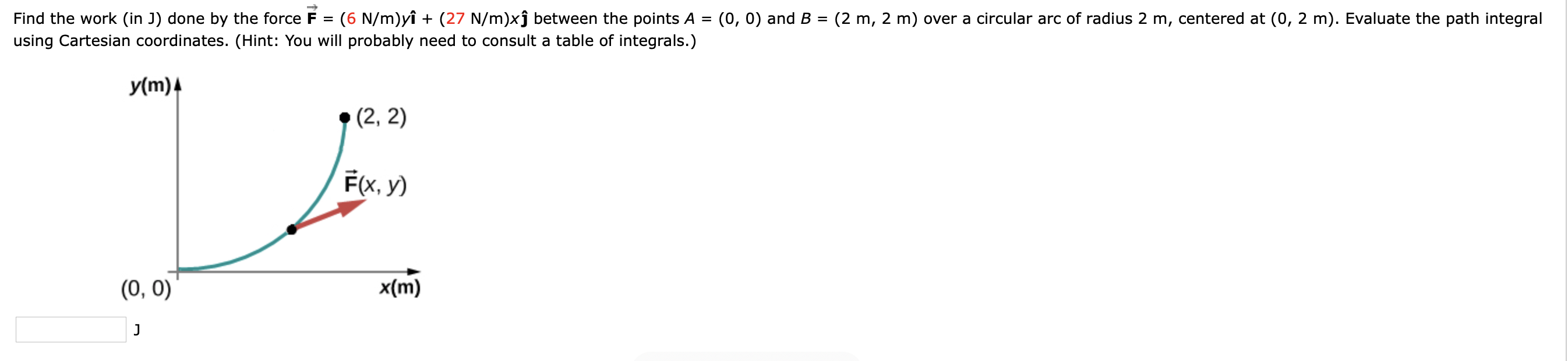# Find the work (in J) done by the force F (6 N/m)yî + (27 N/m)xĵ between the points A = (0, 0) and B = (2 m, 2 m) over a circular arc of radius 2 m, centered at (0, 2 m). Evaluate the path integr %3D using Cartesian coordinates. (Hint: You will probably need to consult a table of integrals.) y(m)4 (2, 2) F(x, y) (0, 0) x(m)

Questionhelp_outlineImage TranscriptioncloseFind the work (in J) done by the force F (6 N/m)yî + (27 N/m)xĵ between the points A = (0, 0) and B = (2 m, 2 m) over a circular arc of radius 2 m, centered at (0, 2 m). Evaluate the path integr %3D using Cartesian coordinates. (Hint: You will probably need to consult a table of integrals.) y(m)4 (2, 2) F(x, y) (0, 0) x(m) fullscreen

1 Rating

### Want to see this answer and more?

Experts are waiting 24/7 to provide step-by-step solutions in as fast as 30 minutes!*

*Response times may vary by subject and question complexity. Median response time is 34 minutes for paid subscribers and may be longer for promotional offers.
Tagged in
Science
Physics

### Other